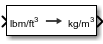# Density Conversion

Convert from density units to desired density units

• Library:
• Aerospace Blockset / Utilities / Unit Conversions

•## Description

The Density Conversion block computes the conversion factor from specified input density units to specified output density units and applies the conversion factor to the input signal.

The Density Conversion block port labels change based on the input and output units selected from the Initial unit and the Final unit lists.

## Ports

### Input

expand all

Contains the density, specified as a scalar or array, in initial density units.

#### Dependencies

The input port label depends on the Initial unit setting.

Data Types: `double`

### Output

expand all

Contains the density, returned as a scalar or array, in initial density units.

#### Dependencies

The output port label depends on the Final unit setting.

Data Types: `double`

## Parameters

expand all

Input units, specified as:

 `lbm/ft^3` Pound mass per cubic foot `kg/m^3` Kilograms per cubic meter `slug/ft^3` Slugs per cubic foot `lbm/in^3` Pound mass per cubic inch

#### Dependencies

The input port label depends on the Initial unit setting.

#### Programmatic Use

 Block Parameter: `IU` Type: character vector Values: `'lbm/ft^3'` | `'kg/m^3'` | `'slug/ft^3'` | `'lbm/in^3'` Default: `'lbm/ft^3'`

Output units, specified as:

 `lbm/ft^3` Pound mass per cubic foot `kg/m^3` Kilograms per cubic meter `slug/ft^3` Slugs per cubic foot `lbm/in^3` Pound mass per cubic inch

#### Dependencies

The output port label depends on the Final unit setting.

#### Programmatic Use

 Block Parameter: `OU` Type: character vector Values: `'lbm/ft^3'` | `'kg/m^3'` | `'slug/ft^3'` | `'lbm/in^3'` Default: `'kg/m^3'`

## Extended Capabilities

### C/C++ Code GenerationGenerate C and C++ code using Simulink® Coder™.